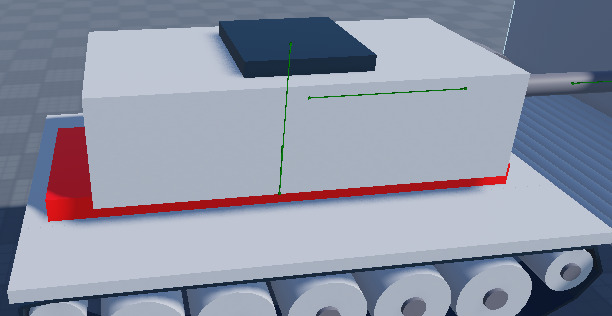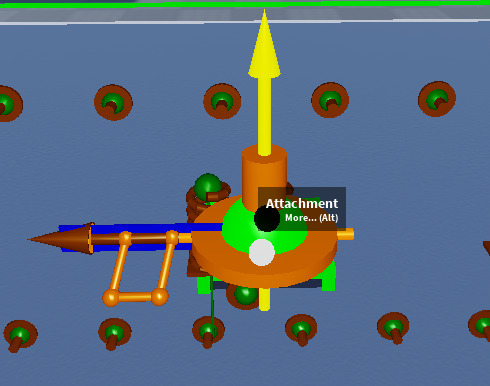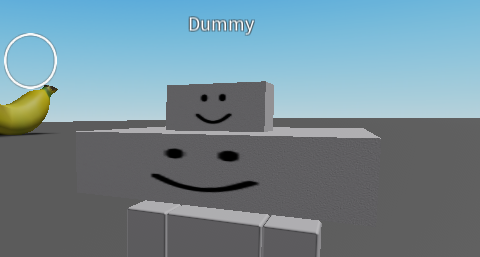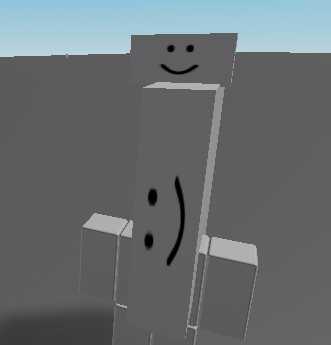# Gun rotation messed up when changing rotation of base

im making a tank and got everything working except when i turn its messed up

code:

``````local mouse = plr:GetMouse()
local delta =  mouse.Hit.Position - rotateOrigin.Position

turretBase.TargetAngle = math.deg(math.atan2(delta.Z, -delta.X))
``````red part is rotateOriginmain hinge (orange arrow is pointing forward)

video:

1 Like

Try this to make it relative to the base of the turret.

``````local delta =  mouse.Hit.Position - rotateOrigin.Position
local deltaRelativeToBase = rotateOrigin.CFrame:PointToObjectSpace(delta)
turretBase.TargetAngle = math.deg(math.atan2(deltaRelativeToBase .Z, -deltaRelativeToBase .X))
``````

Try using the attachments world CFrame as the base CFrame

``````local delta =  mouse.Hit.Position - rotateOrigin.Position
local deltaRelativeToBase = turretBase.Attachment0.WorldCFrame:PointToObjectSpace(delta)
turretBase.TargetAngle = math.deg(math.atan2(deltaRelativeToBase .Z, -deltaRelativeToBase .X))
--or maybe
local delta =  mouse.Hit.Position - rotateOrigin.Position
local deltaRelativeToBase = turretBase.Attachment1.WorldCFrame:PointToObjectSpace(delta)
turretBase.TargetAngle = math.deg(math.atan2(deltaRelativeToBase .Z, -deltaRelativeToBase .X))
``````

Otherwise try this other calculation method to calculate the angles

neither worked
the first one is off by 90 degress and adding or subtracting 90 just makes it stay facing forward and the second one kept it facing forward

Alright one possible problem is that this heavily depends on how you rigged your turret base.

The calculations assume this that the base initially has the same CFrame as the top oneIt will be different if the base is rotated, where the yaw angle becomes the pitch angle as the axises become different according to CFrame math since it’s relative to base so the head in this case is rotated 90 degrees in the Z axis and will cause errors.The reason why I gave you the code bit taken from ThanksRoBama code:

Perhaps Sleitnicks method is better because you can choose the vectors from which you want to measure the angle:

1 Like

so it works if i dont move my camera and leave it in a certain area but moving it out of there or moving my camera it breaks

``````-- rotateOrigin is now the gunBase (the big block connecting the barrel)
local targetPosition = mouse.Hit.Position
local turretPosition = rotateOrigin.Position
local turretLookVector = rotateOrigin.CFrame.LookVector -- this is facing the barrel
local targetLookVector = (targetPosition - turretPosition).Unit

local axis = Vector3.new(0, 1, 0)
local angle = AngleBetweenSigned(turretLookVector, targetLookVector, axis)
turretBase.TargetAngle = math.deg(angle)
``````
``````local offset = rotateOrigin.CFrame:PointToObjectSpace(mouse.Hit.Position)
local angle = math.atan2(-offset.X, -offset.Z)
turretBase.TargetAngle = math.deg(angle)
``````

after changing the base of the turret to make the lookvector correct this worked

3 Likes

Got the same exact issue too, can you elaborate on “changing the base of the turret to make the lookvector”? Using the same script as you too.

the lookvector being the front face of the part